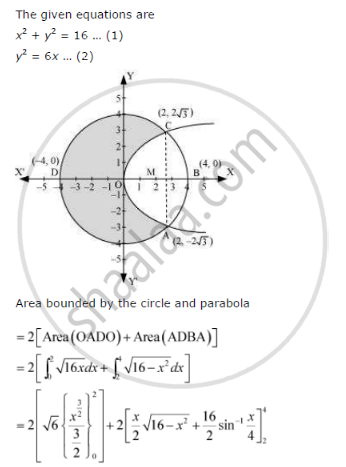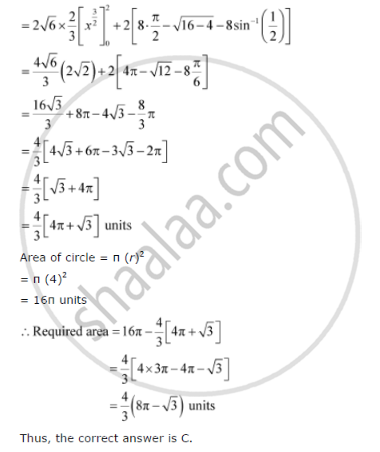Share

# The Area of the Circle X2 + Y2 = 16 Exterior to the Parabola Y2 = 6x Is - CBSE (Commerce) Class 12 - Mathematics

#### Question

Choose the correct answer The area of the circle x2 + y2 = 16 exterior to the parabola y2 = 6x is

A. 4/3 (4pi - sqrt3)

B. 4/3 (4pi + sqrt3)

C. 4/3 (8pi - sqrt3)

D.4/3 (4pi + sqrt3)

#### SolutionIs there an error in this question or solution?

#### APPEARS IN

NCERT Solution for Mathematics Textbook for Class 12 (2018 to Current)
Chapter 8: Application of Integrals
Q: 18 | Page no. 376

#### Video TutorialsVIEW ALL 

Solution The Area of the Circle X2 + Y2 = 16 Exterior to the Parabola Y2 = 6x Is Concept: Area Between Two Curves.
S